# 干货 | 滴滴 数据分析原来是这样做的！

08/06 20:00

hi，我是 Rilke YangA/B测试是互联网企业常用的一种基于数据的产品迭代方法，它的主要思想是在控制其他条件不变的前提下对不同（或同一、同质）样本设计不同实验水平（方案），并根据最终的数据变现来判断自变量对因变量的影响；A/B测试的理论基础主要源于数理统计中的假设检验部分，此部分统计学知识读者可自行探索。

#### 数据说明

test.xlsx city.xlsx
date：日期 date：日期
group：组别（控制组/实验组） hour：时点
requests：订单请求数 requests：请求数
gmv：成交总额 trips：订单数
coupon per trip：每单优惠券金额 supply hours：可服务时长
trips：订单数 average minutes of trips：平均订单时长（分钟）
canceled requests：取消请求数 pETA：顾客预计等待时长
aETA：顾客实际等待时长
utiliz：司机在忙率

test.xlsx 数据可以用来判断实验条件对此次A/B测试的结果影响是否显著；city.xlsx 数据可以用来探索该城市运营中出现的问题，根据关键结论辅助决策。

## 一、A/B测试效果分析

### 1、数据导入

``````#A/B测试结果数据导入

import pandas as pd

``````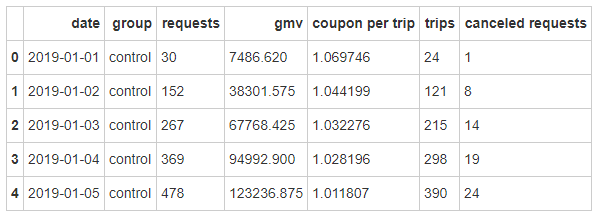### 2、计算ROI

``````#计算优惠券投入相对gmv的ROI

test['ROI']=test['gmv']/(test['coupon per trip']*test['trips'])
``````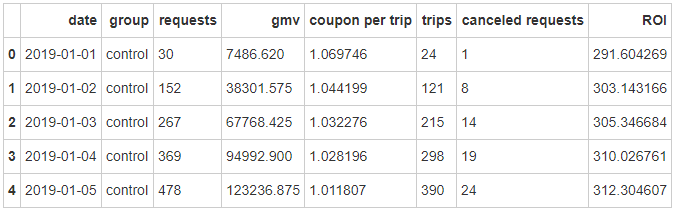### 3、requests检验

#### 3.1 requests方差检验

1. 记两组requests方差分别为从c1,c2

2. 零假设H0：c1=c2；备选假设：H1：c1≠c2

3. 显著性水平取0.05

``````#levene检验requests是否齐方差

requests_A=test[test.group=='control'].requests
requests_B=test[test.group=='experiment'].requests

import scipy.stats as st
st.levene(requests_A,requests_B)
``````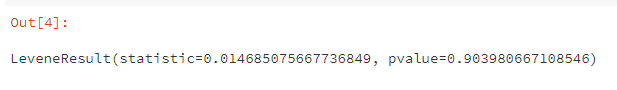p值大于0.05，不拒绝原假设，因此可认为两组实验requests齐方差。

#### 3.2 requests均值检验

1. 该数据为同一样本实验前后的不同水平，因此选用配对样本t检验。

2. 记两组requests均值分别为从u1,u2

3. 零假设H0：u1=u2；备选假设：H1：u1≠u2

4. 显著性水平取0.05

``````#配对样本t检验（两独立样本t检验之前需检验是否齐方差，此处不需要）

st.ttest_rel(requests_A,requests_B)
``````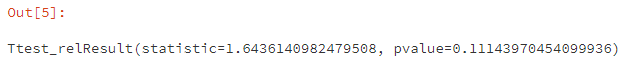p值大于0.05，不拒绝原假设，因此可认为实验条件对requests影响不显著。

### 4、gmv检验

#### 4.1 gmv方差检验

``````#levene检验gmv是否齐方差

gmv_A=test[test.group=='control'].gmv
gmv_B=test[test.group=='experiment'].gmv

st.levene(gmv_A,gmv_B)
``````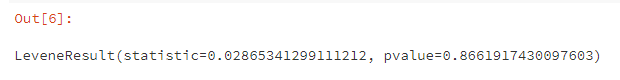p值大于0.05，不拒绝原假设，因此可认为两组实验gmv齐方差。

#### 4.2 gmv均值检验

``````#配对样本t检验（两独立样本t检验之前需检验是否齐方差，此处不需要）

st.ttest_rel(gmv_A,gmv_B)
``````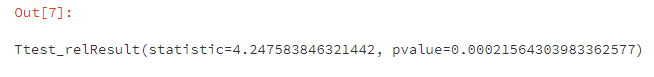p值小于0.05，拒绝原假设，因此可认为实验条件对gmv有显著影响。

### 5、ROI检验

#### 5.1 ROI方差检验

``````#levene检验ROI是否齐方差

ROI_A=test[test.group=='control'].ROI
ROI_B=test[test.group=='experiment'].ROI

st.levene(ROI_A,ROI_B)
``````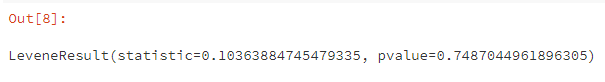p值大于0.05，不拒绝原假设，因此可认为两组实验ROI齐方差。

#### 5.2 ROI均值检验

``````#配对样本t检验（两独立样本t检验之前需检验是否齐方差，此处不需要）

st.ttest_rel(ROI_A,ROI_B)
``````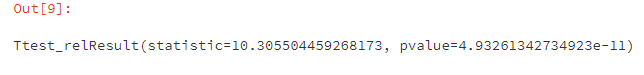p值小于0.05，拒绝原假设，因此可认为实验条件对ROI有显著影响。## 二、城市运营分析

### 1、数据导入

``````#导入该城市运营相关数据

``````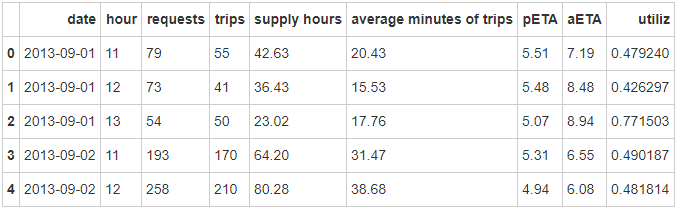``````#查看数据有无缺失值

city.info()
``````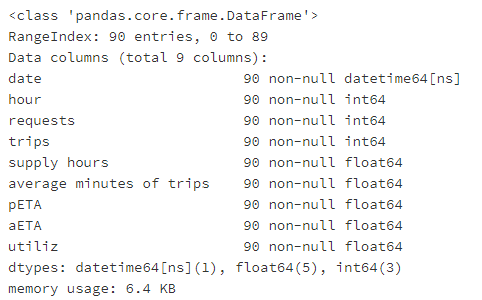### 2、数据探索

#### 2.1 单量最多的时间点

``````req_hour = city.groupby(['hour'],as_index=True).agg({'requests':sum},inplace=True)
req_hour
``````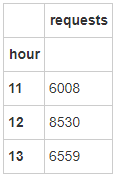``````#绘制各时点订单请求柱状图

import matplotlib.pyplot as plt

req_hour.plot(kind='bar')
plt.xticks(rotation=0)

plt.show()
``````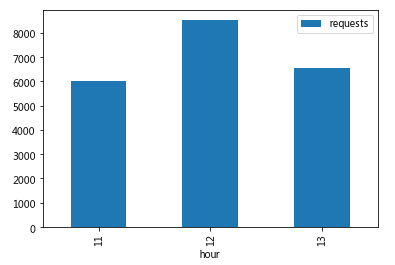#### 2.2 单量最多的日期

``````req_date = city.groupby(['date'],as_index=True).agg({'requests':sum},inplace=True)
``````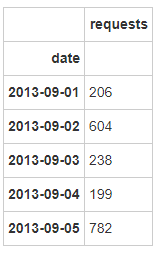``````#绘制订单请求数随日期变化的折线图

req_date.plot(kind='line')

plt.show()
``````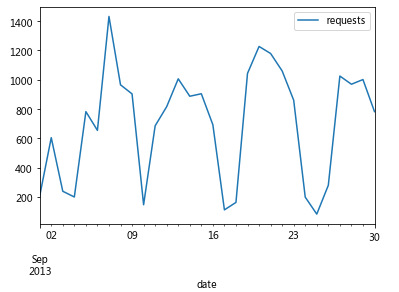#### 2.3 各时段订单完成率

``````com_hour = city.groupby(['hour'],as_index=False).agg({'requests':sum,'trips':sum},inplace=True)
com_hour['rate']=com_hour['trips']/com_hour['requests']
com_hour
``````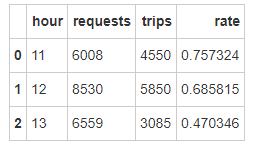13点订单需求较多，但订单完成率仅47%，说明较多订单没有得到及时相应。

#### 2.4 单月每日订单完成率

``````com_date = city.groupby(['date'],as_index=True).agg({'requests':sum,'trips':sum},inplace=True)
com_date['rate']=com_date['trips']/com_date['requests']
``````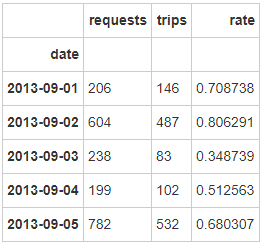``````#绘制订单完成率随日期变化的折线图

com_date.rate.plot(kind='line')

plt.show()
``````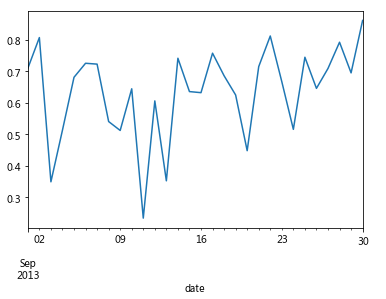#### 2.5 顾客等待时间

``````import numpy as np

eta_hour = city.groupby(['hour'],as_index=True).agg({'pETA':np.mean,'aETA':np.mean},inplace=True)
eta_hour
``````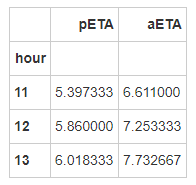``````#绘制顾客等待时长复合柱状图

eta_hour.plot(kind='bar')
``````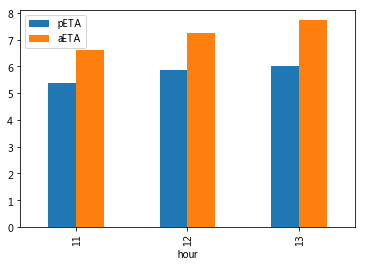#### 2.6 司机在忙率

``````city['busy'] = city['supply hours']*city['utiliz']
``````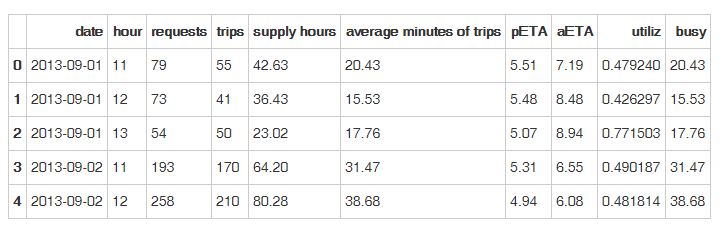``````busy_hour = city.groupby(['hour'],as_index=False).agg({'supply hours':sum,'busy':sum})
busy_hour['utiliz'] = busy_hour['busy']/busy_hour['supply hours']
busy_hour
``````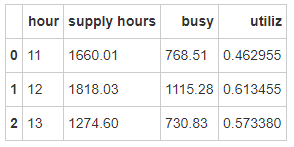12点司机在忙总时长最长，在忙率也最高，用户订单请求也最多，说明车辆总数偏少。

#### 2.7 订单时长

``````trip_min = city.groupby(['hour'],as_index=False).agg({'average minutes of trips':np.mean})
trip_min
``````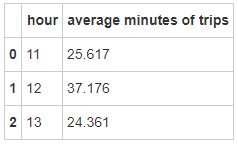12点用户订单需求较多，同时订单时长最长，说明这个时间点是一个非常重要的时间点。

``````supply_hour = city.groupby(['hour'],as_index=False).agg({'supply hours':np.mean})
supply_hour
``````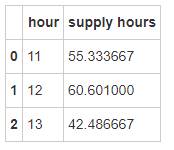13点订单量也较大，此时点司机服务时长较短。

### 3、后续思考方向：

• 提升顾客预计等待时长预测准确度（需要历史数据进行预测）

• 加大车辆投入（分车辆不同等级来看，因此可能需要车辆相关信息表）

• 优化用户体验（需要客诉相关数据）

• 优化平台派单逻辑（需要订单的位置相关数据）

• 个性化需求（需要用户属性、及其他行为数据）

https://alltodata.cowtransfer.com/s/9bb9acdc15ae40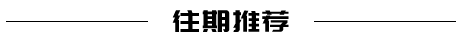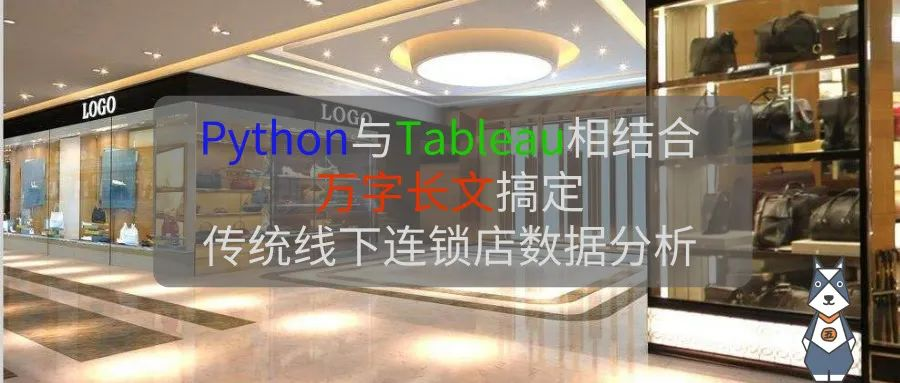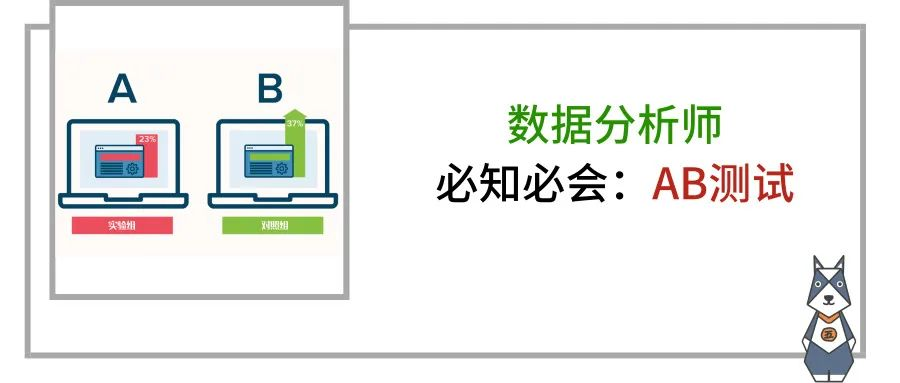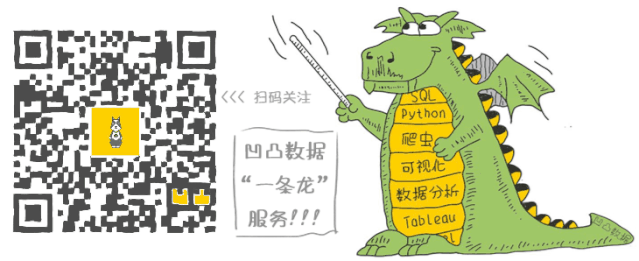PS  当当新用户优惠码：DPC3CX

1
18 收藏

### 作者的其它热门文章"精通excel" ☑达成
08/20 13:00

👍数据分析师
08/20 10:38

2 评论
18 收藏
1